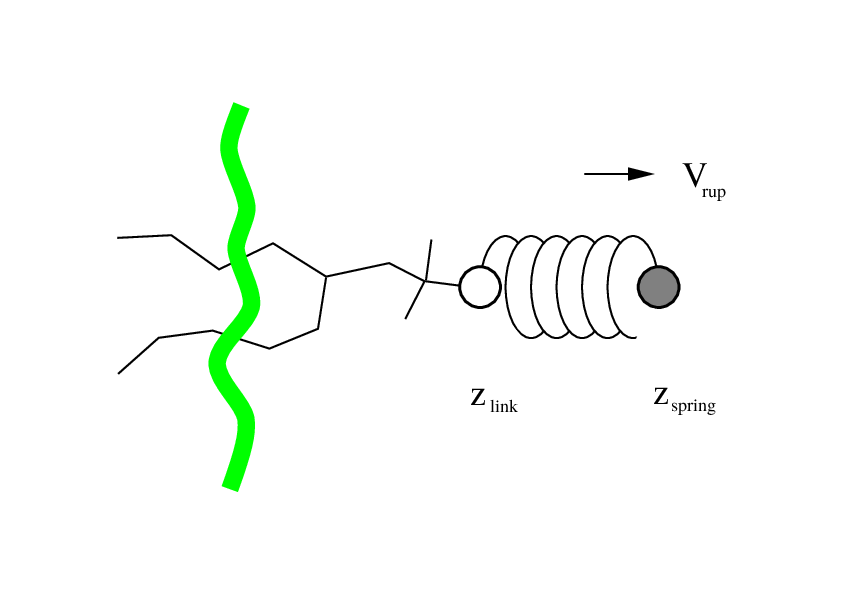# Extending Non-Equilibrium Pulling Method in GROMACS with Arbitrary User-Defined Atom Weight Factor Expressions

## Outline

• Molecular dynamics simulation
• Non-equilibrium pulling
• Uniform flow simulation
• Atom weight factor expression
• Applications

## Molecular dynamics simulation

• Simulation of physical movement of atoms and molecules
• Trajectories of atoms and molecules are obtained by solving Newton's equations of motion many times
• Typical time step: 1-2 femtoseconds
• Typical simulated time: nanoseconds to microseconds (10^6 to 10^9 steps)

## Non-equilibrium pulling## Pull coordinate

• Pull coordinate = distance, angle, or dihedral angle betwen centers of mass of two groups of atoms
• Alternatively, one group can be replaced by a reference position in space
• Combination of coordinates using (optionally time-dependent) expressions: transformation pull coordinate, e.g. 0.5*(x1 + x2)

## Pull groups and force

• Pull group = a group of atoms weighted by their mass
• Aditional per-atom weighting factor can be used
• Defined in the simulation parameters, remains the same during simulation
• Constant force pulling applies constant force between the centers of mass of two groups

## Processes activated by flow

• Many biological processes occur under flow conditions, e.g.
• Hemostasis to stop bleeding
• Leukocyte adhesion at sites of inflammation
• Spider silk spinning
• The biomolecules involved in the flow-dependent processes undergo specific conformational changes triggered (or influenced) by the flow

## Simulating uniform flow (1/3)## Simulating uniform flow (2/3)

• Flow is a movement of solvent atoms
• Pulling all solvent atoms works when no other molecules except water are present in the simulation
• Just a slice of solvent molecules should be pulled to allow the solvent atoms to interact with the biomolecule(s) without being "dragged away"

## Simulating uniform flow (3/3)## The previous approach (1/4)

• This approach is flow-specific
• Uses atom weighting factors in GROMACS to non-uniformly distribute the pulling force
• Atoms of the solvent inside the slice -> weighting factor is non-zero
• Atoms of the solvent outside the slice -> weighting factor is zero

## The previous approach (2/4)

• GROMACS limitation: weighting factors are defined once at the begining of the simulation
• Problem: solvent atoms move during flow simulation, weighting factors should be recomputed each step
• Solution: hack GROMACS to introduce the concept of the slice and recompute atom weighting factors each step

## The previous approach (3/4)

• Each atom is weighted with the weight factor:

$w_{sp} = x_{unit}^4 - 2x_{unit}^2 + 1$
• Coordinate $$x$$ of each atom in the arbitrary slice is normalized from the interval $$[s_{min}, s_{max}]$$ to the coordinate $$x_{unit}$$ in the interval $$[−1, 1]$$:

$x_{unit} = \frac{x - \frac{s_{min} + s_{max}}{2}}{\frac{s_{min} - s_{max}}{2}}$

## The previous approach (4/4)## The new, general approach (2/3)

• Weight factor expression variables:
• Atom position in 3D (x, y, z)
• Atom velocity in 3D (vx, vy, vz)
• Examples:
• Atom weight factor is sum of squares of positions: x^2 + y^2 + z^2
• Atom weight factor is a linear combination of velocities: 1.75 * vx + 1.5 * vy + 1.25 * vz

## The new, general approach (3/3)

• Covers the requirements of uniform flow simulation
• Non-smooth slice: x >= 1 && x < 5 ? 1 : 0
• Smooth slice: x >= 1 && x < 5 ? (x - 2)^4 - 2 * (x - 2)^2 + 1 : 0

## Possible applications of atom weight factor expressions

• Simulating uniform flow in arbitrary direction
• Pulling a protein domain with a non-uniform force applied to different residues
• Introducing external force in a subset of the periodic metal/material system

## Future plans

• Providing the option to configure the number of steps when weight factors are recomputed
• Adding heuristics that avoid recomputation of factors for atoms that did not move or accelerate very much
• Adding time as a variable in the expression
• More extensive testing and physical validation
• Inclusion in a future version of GROMACS (2024?)

## Acknowledgments## GROMACS developer workshop

• Planned ~= spring 2023 (time and location TBA)
• Entry-level development workshop
• Primarily targeted for new developers
• Non-developers interested in exploring development are welcome
• Follow GROMACS forums or @GMX_TWEET for announcements

Author: Vedran Miletić